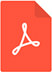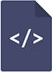# Introduction of explicit equations for the estimation of surface tension, specific weight, and kinematic viscosity of water as a function of temperaturePDF Full Text

# Abstract

Three simple empirical models were proposed to predict surface tension, specific weight, and kinematic viscosity of water as a function of temperature. The formulations were derived within the framework of the nonlinear regression analysis based on the Richardson’s extrapolation method and the Levenberg–Marquardt algorithm. The estimations were proven to be satisfactory with very high determination coefficients above 0.999. The proposed formulations were developed using a total of 155 data points and compared against different equations from the literature. Moreover, illustrative examples and relevant MATLAB® scripts were presented to demonstrate the applicability of the present equations. The statistical results clearly corroborated that the proposed equations were accurate enough to be used in estimation of the present fluid mechanics-related parameters. The computational analysis yielded simple mathematical structures to be easily used for educational and practical purposes.

# Keywords

fluid mechanics, surface tension, specific weight, kinematic viscosity, water, temperature, nonlinear regression, statistical analysis